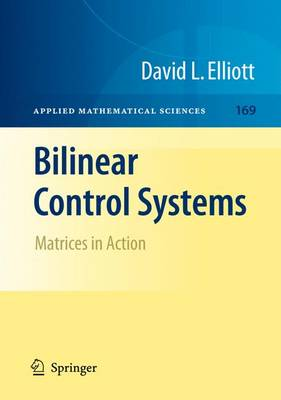•# Bilinear Control Systems: Matrices in Action - Applied Mathematical Sciences 169 (Paperback)

(author)
£74.99
Paperback 281 Pages / Published: 19/10/2010
• We can order this from the publisher

Usually dispatched within 15 working days

The mathematical theory of control became a ?eld of study half a century ago in attempts to clarify and organize some challenging practical problems and the methods used to solve them. It is known for the breadth of the mathematics it uses and its cross-disciplinary vigor. Its literature, which can befoundinSection93ofMathematicalReviews,wasatonetimedominatedby the theory of linear control systems, which mathematically are described by linear di?erential equations forced by additive control inputs. That theory led to well-regarded numerical and symbolic computational packages for control analysis and design. Nonlinear control problems are also important; in these either the - derlying dynamical system is nonlinear or the controls are applied in a n- additiveway.Thelastfourdecadeshaveseenthedevelopmentoftheoretical work on nonlinear control problems based on di?erential manifold theory, nonlinear analysis, and several other mathematical disciplines. Many of the problems that had been solved in linear control theory, plus others that are new and distinctly nonlinear, have been addressed; some resulting general de?nitions and theorems are adapted in this book to the bilinear case.

Publisher: Springer
ISBN: 9789048181698
Number of pages: 281
Weight: 450 g
Dimensions: 235 x 155 x 15 mm
Edition: Softcover reprint of hardcover 1st ed. 2009

MEDIA REVIEWS
From the reviews:

"... in spite of their intrinsic importance, there are relatively few treatments of bilinear control systems in monograph form that are accessible to students; that is why the book under review is a welcome addition to the control-theory literature...As with any good introductory treatment, the author smartly avoids trying to be encyclopedic and getting bogged down in peripheral technical minutia...

A unique feature of the book is the inclusion of a half-dozen Mathematica scripts that aid in explicit computations of such tasks as finding a basis for the matrix Lie algebra generated by the bilinear system's matrices B1, . . . ,Bm and determining the generic Lie rank of a finite collection of linear vector fields. This book is highly recommended to both students and researchers in control theory."Kevin A. Grasse, MathSciNet, 2009

"This work by Elliott ... is a textbook for a graduate course in a subarea of control theory. ... The work provides an extensive reference section to the literature of the field. It will be useful to libraries supporting graduate programs in engineering with courses in control theory, and will also be valuable to researchers in disciplines that use control theory. Summing Up: Recommended. Graduate students, researchers, faculty, and practitioners." (D. Z. Spicer, Choice, Vol. 47 (3), November, 2009)

"The monograph deals with problems of nonlinear systems analysis. The author examines bilinear control systems as time-invariant nonlinear systems with control inputs that appear as coefficients. ... Examples of bilinear control systems have been given in a separate chapter. ... Main results are illustrated by examples, proofs are given or cited. Appendices A-D contain standard facts about matrices, Lie groups and Lie algebras that are helpful for readers." (Valeriy N. Tkachenko, Zentralblatt MATH, Vol. 1171, 2009)